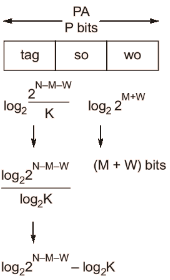# GATE Solved Paper 2017-19 - GATE 2018

• A

Hi Bye Bye Hi• B

Hi Bye Hi Bye• C

Bye Hi Hi Bye• D

Bye Hi Bye Hi• Option : A
• Explanation :
fun1(char *s1, char *s2)
function scope is local, so the value changed So the affect actual parameters. SO the values will be ‘Hi Bye’.
fun2(char **s1, char **s2)
In this function value is pointer to pointer, so it changes pointer of the actual value. So values will be ‘Bye Hi’

• Option : A
• Explanation :
∀ are always True and ∃ are always False for empty sets.
So there exists at least one model with universe of size 3 (or less than). Therefore, option (A) is necessarily TRUE.

• A

I only• B

II only• C

Both I and II• D

Neither I nor II• Option : A
• Explanation :
For statement (II) take counter example of complete graph of three vertices, i.e., K3 with XYZ, where X is source and Y and Z are in the same level. Also, there is an edge between vertices Y and Z, i.e., |i-j| = 0 ≠ 1 in BFS. So, statement became false. Option (A) is correct.

• A

P−N−log2K• B

P−N+log2K• C

P−N−M−W−log2K• D

P−N−M−W+log2K• Option : B
• Explanation :
MM space = 2P bytes
Physical Address (PA) size = P bits
CM size = 2N bytes
Block size 2M words
2M words*2W bytes/word
2M+W bytes
Number of lines = (CM size)/Block size ⇒ 2N/(2M+W)
⇒ 2N-M-W
Number of sets = (Number in cm)/P-way
= 2N-M-W/K⇒ (N-M-W-log2K)
∴ Tag size
⇒ P-(N-M-W-log2K)
⇒ P-N+log2K)

• A

Only I and II are undecidable• B

Only III is undecidable• C

Only II and IV are undecidable• D

Only I, II and III are undecidable• Option : D
• Explanation :
Here the match flowing.
I. Membership problem for RE → undecidable
II. Regularity problem for RE → undecidable
III. Equivalence problem for RE → undecidable
IV. Since DPDA P exists for every nfa N and equivalent to it, this problem is trivially decidable.
Related Quiz.
GATE 2018